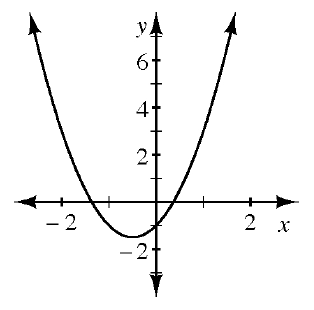### Home > CCAA8 > Chapter 8 Unit 9 > Lesson CCA: 8.2.4 > Problem8-94

8-94.Examine the graph of $y=2x^2+2x-1$ at right.

1. Estimate the zeros $y=2x^2+2x-1$ from the graph.

Where are the $x$-intercepts of this graph?

2. What happens if you try to use the Zero Product Property to find the roots of $2x^2+2x-1=0$?

What makes the Zero Product Property difficult to use here?